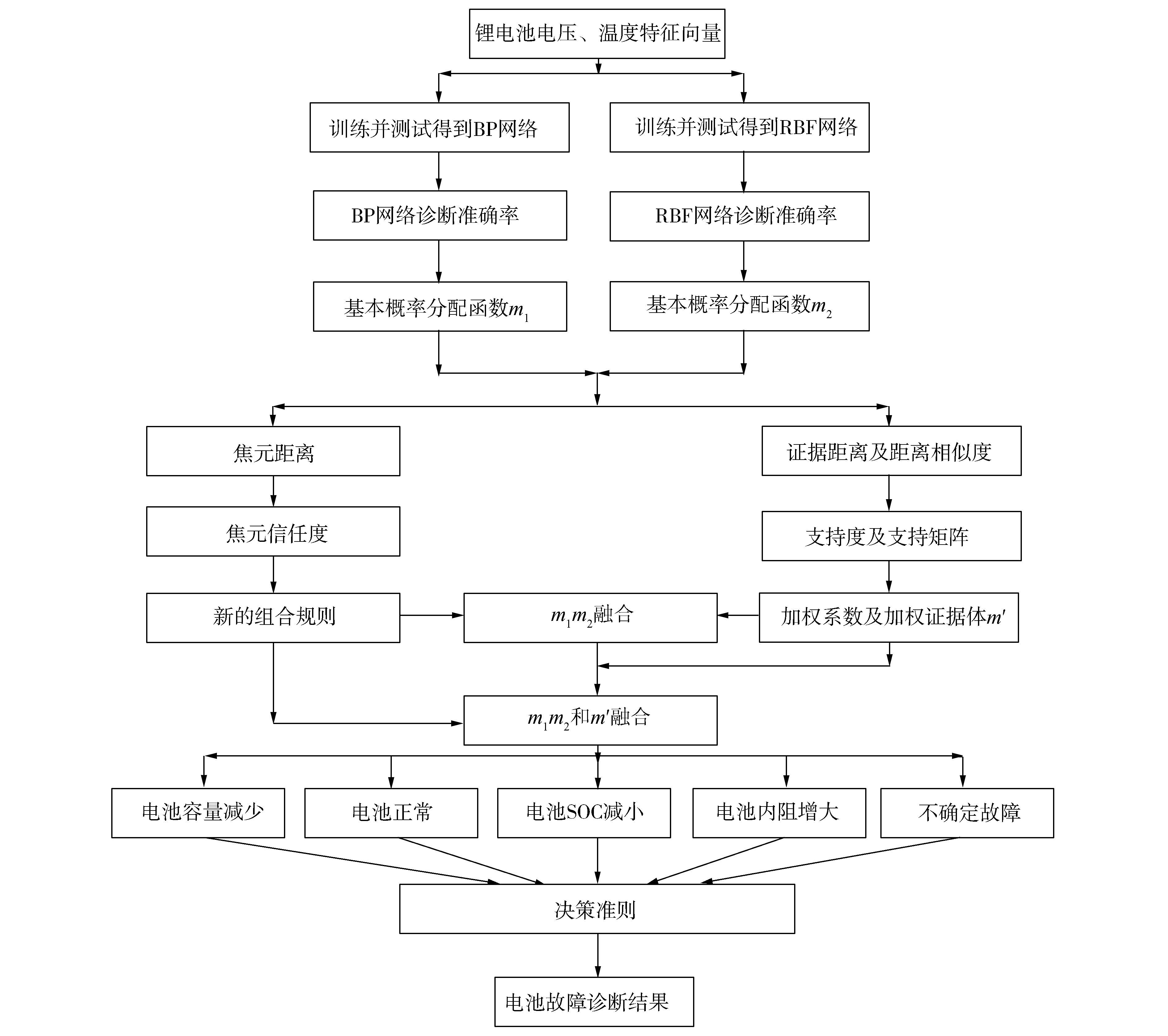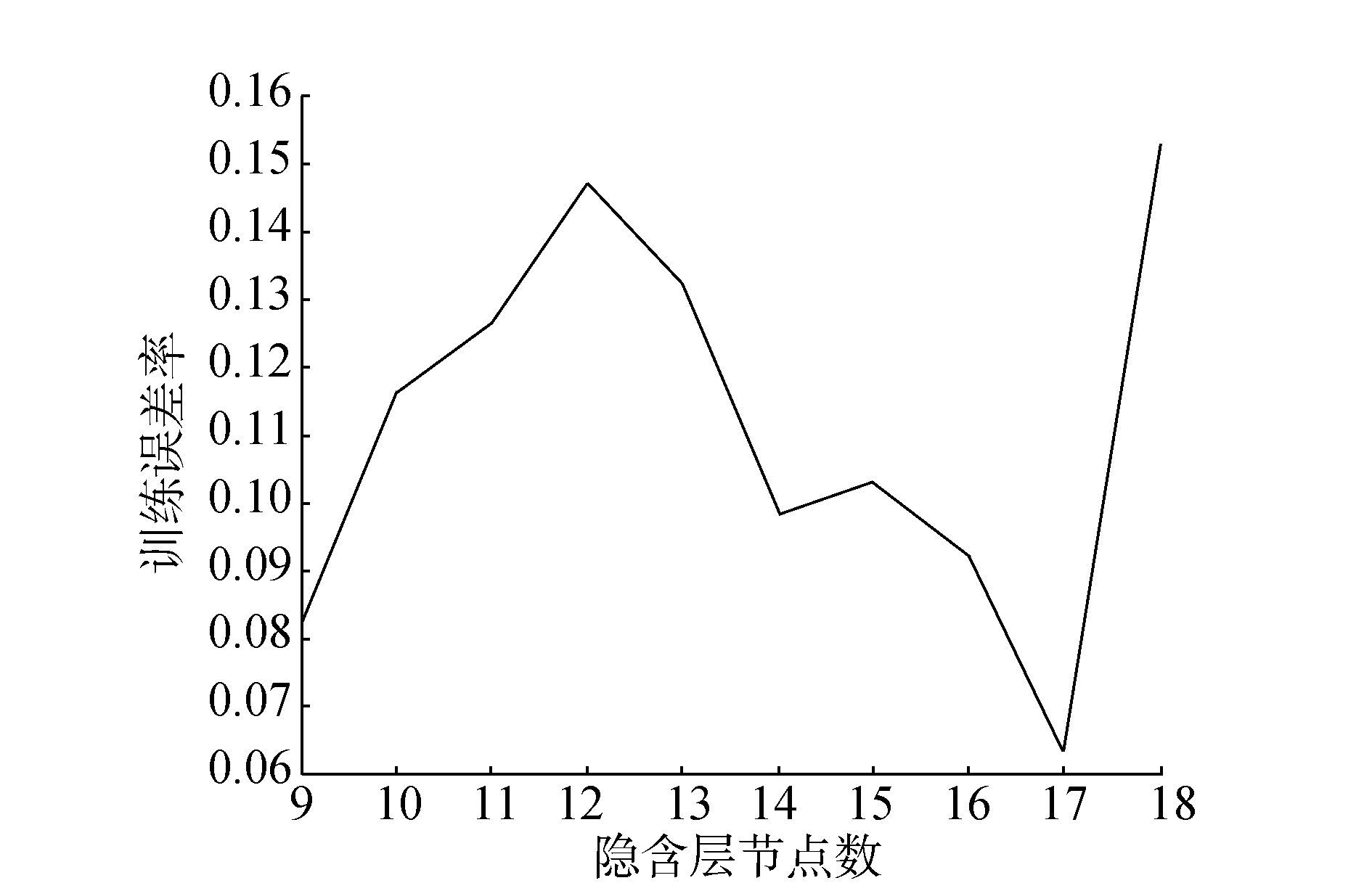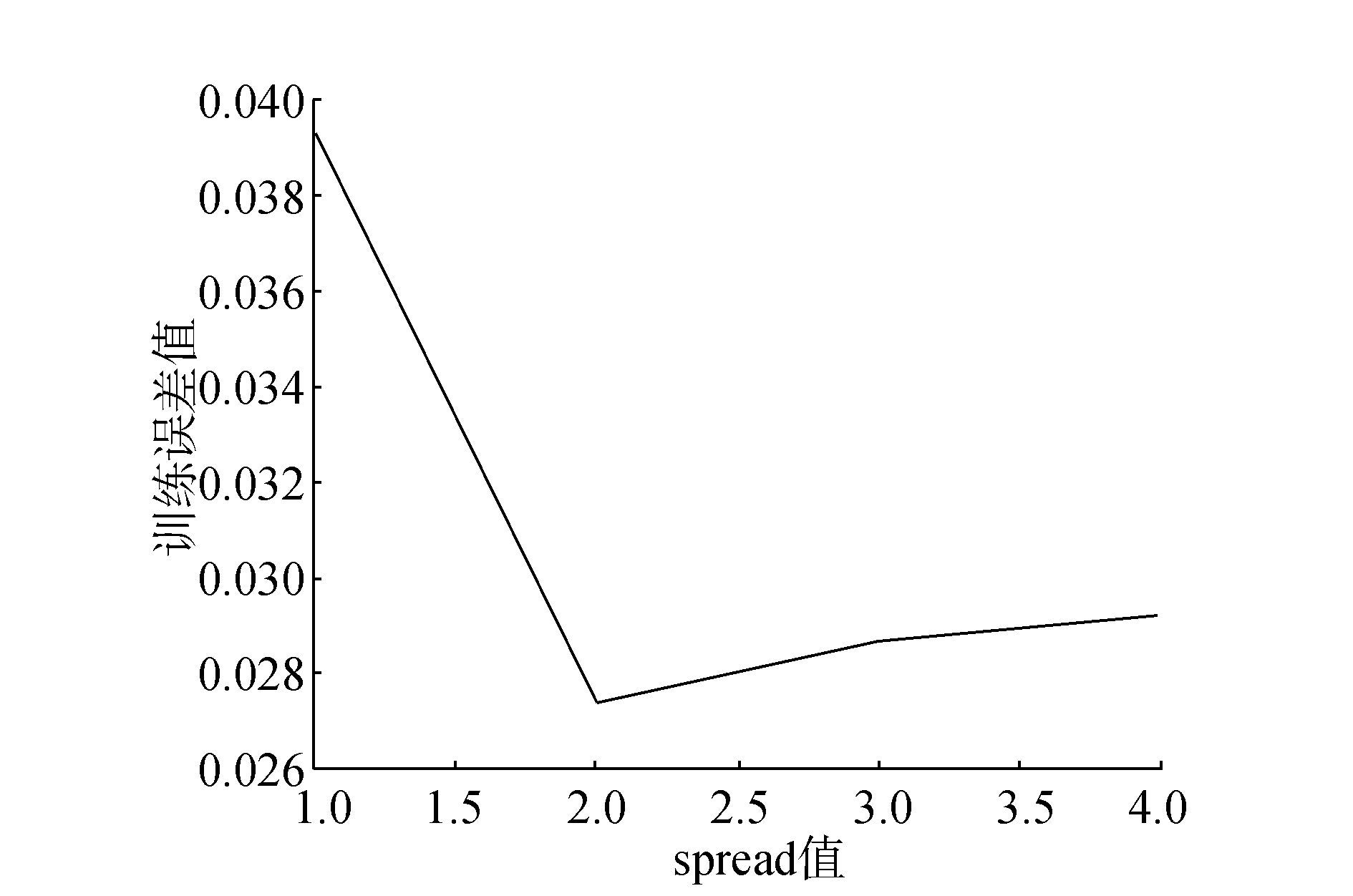﻿ 改进D-S证据理论在电动汽车锂电池故障诊断中的应用
«上一篇文章快速检索 高级检索

 智能系统学报2017, Vol. 12Issue (4): 526-537  DOI: 10.11992/tis.2016050010

### 引用本文XIA Fei, MA Xi, ZHANG Hao, et al. Application of improved D-S evidence theory in fault diagnosis of lithium batteries in electric vehicles[J]. CAAI Transactions on Intelligent Systems, 2017, 12(4): 526-537. DOI: 10.11992/tis.201605001.### 文章历史

1. 上海电力学院自动化工程学院, 上海 200090;
2. 上海发电过程智能管控工程技术研究中心, 上海 200090;
3. 同济大学电子与信息工程学院, 上海 201804

Application of improved D-S evidence theory in fault diagnosis of lithium batteries in electric vehicles
XIA Fei1,2,3, MA Xi1,2, ZHANG Hao1,2,3, PENG Daogang1,2, SUN Peng1,2, LUO Zhijiang1,2
1. College of Automation Engineering, Shanghai University of Electric Power, Shanghai 200090, China;
2. Shanghai Engineering Research Center of Intelligent Management and Control for Power Process, Shanghai 200090, China;
3. College of Electronics and Information Engineering, Tongji University, Shanghai 201804, China
Abstract: In this study, we used the improved Dempster-Shafer (D-S) evidence theory combination rules based on the neural network to construct a fault diagnosis process for an electric vehicle battery system.To avoid misdiagnoses and missed diagnoses caused by a single fault diagnosis method, we applied the D-S evidence theory combination principle to determine the result based on the back-propagation (BP) network and radial basis function (RBF) network fault diagnosis algorithm.However, to overcome the defects in the D-S evidence theory in dealing with highly conflicting evidence, we propose a D-S evidence theory combination principle based on an improved neural network.First, we apply a neural network to perform a preliminary diagnosis regarding battery failure and the accuracy of the network diagnosis.Then, we distribute indefinite information and construct a body of evidence.We also introduce a support matrix of this evidence to determine a new weighted body of evidence.We then integrate the credibility of every focal element into the D-S evidence theory combination rules to fuse the neural network body of evidence with the new weighted body of evidence.Lastly, based on the decision criterion, we determine the failure state of the lithium battery system.Our simulation results show that our proposed improved D-S evidence theory fusion diagnosis method is effective in the fault diagnosis of electric vehicles with lithium batteries.
Key words: fault diagnosis    electric vehicle    lithium battery    improved evidence theory    information fusion

1）对数据模型的修改。由于传感器处于外界环境，经常受到周围自然环境、人为因素的干扰以及传感器自身性能的影响，常常需要对传感器得到的证据数据修改其权重信息。目前对证据修正其权重可以快速有效地识别出系统存在的冲突证据。通常是利用证据距离函数表达证据体之间的距离及相似性程度，同时还有证据距离函数和其他函数联合共同修正证据源，包括了证据体的可靠度、方向相似度函数等。利用证据体间贴近度及贴近度概率转换规则的相关系数确定不同的权重因子来修正证据源。文献提出了矛盾系数来确定证据的权重。文献综合了证据体可信度和证据源可靠度给出了证据可信度的计算公式，但是对于两个支持度的权重大小如何选择仍然没有客观的计算方法。这些改进方法仅仅采用证据间每个焦元被赋予的基本概率分配函数值的差值来衡量证据间的距离，但是没有考虑在某一个证据体中相应焦元之间的关联性，在证据源不确定的情况下，证据之间的距离描述得不够准确。

2）对组合规则的改进。Yager首先提出了冲突信息的分配，将不能起到正面效果的冲突信息赋值给辨识框架，处理方法保守。文献认为冲突信息也具有有用信息，对其进行了利用和分配。这两种方法在处理多个证据与一个证据高度冲突时，使未知项获得较大的支持度，无法做出正确决策。文献依据命题的平均支持度进行加权分配冲突信息，但是该方法只是简单的平均加权整合，降低了有用证据的可信度；文献中对辨识框架下不同目标进行了单独考虑，按照证据关于不同目标的冲突程度进行分配；文献根据部分与整体之间的相似性，将每一个命题的BPA的总和看作系统的确定性程度，作为信任度转换的权重。当目标的确定度较大时变换结果应该相对乐观，相反，它还是很保守的。

1 证据理论的改进 1.1 加权证据体生成图 1 基于神经网络的改进D-S证据理论锂电池故障诊断框架图 Fig.1 Improved D-S evidence theory based on neural network Li battery fault diagnosis framework

 $\left\{\begin{array}{l}m_k(A_i)=\frac{C_k(A_i)}{∑\limits^I_{i=1}C_k(A_i)}·R_k,i=1,2,…,I\\m_k(U)=1－∑\limits^I_{i=1}m_k(A_i)\end{array}\right.$ (1)

 $R_k=1－\frac{∑\limits^n_{i=1}{(x_i－y_i)}^2}{∑\limits^n_{i=1}{(x_i－x_v)}^2}$ (2)

1）计算证据体间距离以及距离相似度。辨识框架上相互独立的两个证据体距离和距离相似度可以表示为

 $d_{\text{BPA}}(m_i,m_j)=\sqrt{\frac{1}{2}(m_i－m_j)\mathit{\boldsymbol{D}}(m_i－m_j)}$ (3)
 $\text{DS}(m_i,m_j)=1－d_{\text{BPA}}(m_i,m_j)，i,j=1,2,…,n$ (4)

2）计算证据mi的支持度。证据体mi的支持度表示为

 $\text{sup}(m_i)=∑\limits_{\begin{array}{l}i=1\\j≠i \end{array}}\text{DS}(m_i,m_j)$ (5)

3）计算权重系数。构建证据$E_1,E_2,…,E_n$的支持矩阵$\mathit{\boldsymbol{R}}={(\text{sup}(m_i,m_j))}_{n×n}$

 $β_k=\frac{v_k}{∑\limits^n_{k=1}v_k}，k=1,2,…,n$ (6)

4）利用权重系数对证据进行修正，得到新的证据体：

 $m′=∑\limits^n_{k=1}β(k)×m_k$ (7)

1.2 证据理论组合规则的改进 1.2.1 经典D-S证据理论及改进

 $m(A)= \frac{∑\limits_{A_i∩A_j∩A_l…=A}m_1(A_i)m_2(A_j)m_3(A_l)…}{1－k} A≠\mathit Φ,U$ (8)

 $\left\{\begin{array}{l}m(A)=∑\limits_{A_i∩A_j∩A_l…=A}m_1(A_i)m_2(A_j)m_3(A_l)…\\ m(U)=∑\limits_{A_i∩A_j∩A_l…=\mathit Φ}m_1(A_i)m_2(A_j)m_3(A_l)…=k\end{array}\right.$ (9)

 $\left\{\begin{array}{l}m(A)=\begin{array}{l}∑\limits_{A_i∩B_j∩C_l…=A}m_1(A_i)m_2(A_j)m_3(A_l) …+k·ε·q(A)\ \ A≠\mathit Φ,U\end{array}\\ m(U)=∑\limits_{A_i∩B_j∩C_l...=U}m_1(A_i)m_2(A_j)m_3(A_l)…+k·ε·q(U)+k(1－ε)\end{array}\right.$ (10)

 $\begin{array}{l}m(A)=∑\limits_{A_i∩A_j∩A_l…=A}m_1(A_i)m_2(A_j)m_3(A_l)…+k·q(A)，A≠\mathit Φ,U\end{array}$ (11)

 $\left\{\begin{array}{l}m(\mathit Φ)=0\\m(A)=∑\limits_{A_i∩A_j∩A_l…=A}m_1(A_i)m_2(A_j)m_3(A_l)…+f(A),A≠\mathit Φ,U\end{array}\right.$ (12)

$f(A)=\frac{1}{n}(∑\limits_{A_i∩A_s∩A_k∩…=\mathit Φ}m_1(A_i)m_2(A_s)m_3(A_k)…+$$∑\limits_{A_j∩A_s∩A_k∩…=\mathit Φ}m_2(A_j)m_1(A_s)m_3(A_k)…+…)$，式中：n为证据数，$i,j,k,l,s…=1,2,3,…,n$

1.2.2 改进的D-S证据理论组合规则

 $\left\{\begin{array}{l}m(A)=∑\limits_{A_i∩A_j∩A_l∩…=A}m_1(A_i)m_2(A_j)m_3(A_l)+f(A)\\m(U)=∑\limits_{A_i∩A_j∩A_l∩…=U}m_1(A_i)m_2(A_j)m_3(A_l)\end{array}\right.$ (13)

 $\begin{array}{l}f(A)=∑\limits_{A_i∩A_s∩A_k∩…=\mathit Φ}\frac{D_1(A_i)·m_1(A_i)}{D_1(A_i)·m_1(A_i)+D_2(A_s)·m_2(A_s)+D_3(A_k)·m_3(A_k)+…}\\ ·m_1(A_i)·m_2(A_s)·m_3(A_k)…+\\ ∑\limits_{A_j∩A_s∩A_k∩…=\mathit Φ}\frac{D_2(A_j)·m_2(A_j)}{D_2(A_j)·m_2(A_j)+D_1(A_s)·m_1(A_s)+D_3(A_k)·m_3(A_k)+…}\\ ·m_2(A_j)·m_1(A_s)·m_3(A_k)…+…\end{array}$

 $\begin{array}{l}FD[m_i(A_k)]=\left|m_i(A_k)－m_{AVE}(A_k)\right|\end{array}$ (14)

 $\text{Fcrd}[m_i(A_k)]=[1－\text{FD}[m_i(A_k)]]·\frac{2m_i(A_k)m_{\text{AVE}}(A_k)}{{[m_i(A_k)]}^2+{[m_{\text{AVE}}(A_k)]}^2} i=1,2,…,n;k=1,2,…,K$ (15)
 $\begin{array}{l}D_i(A_k)=\frac{\text{Fcrd}[m_i(A_k)]}{∑\limits^n_{i=1}∑\limits^K_{k=1}\text{Fcrd}[m_i(A_k)]}i=1,2,…,n;k=1,2,…,K\end{array}$ (16)

1.3 决策规则

1）锂电池故障类型存在最大的基本概率分配函数值，即某故障类型的基本概率分配函数输出值是所有故障类型输出值中的最大值。

 $m(A_1)=\text{max}\left\{m(A_i),A_i\subset θ\right\}$ (17)

2）某故障类型与其他故障类型的基本概率分配函数值差异足够大时，才能选择确认锂电池处于该故障状态。

 $\left\{\begin{array}{l}m(A_2)－m(A_1)>ε_1\\m(A_2)=\text{max}\left\{m(A_i),A_i\subset θ,A_i≠A_1\right\}\end{array}\right.$ (18)

3）不确定的基本概率函数值应该小于一定的阈值。

 $m(θ)<ε_2$ (19)

ε1ε2一般根据经验取得，本文取ε1=0.2，ε2=0.5。当融合后的BPA同时满足以上3条决策规则时，可以准确得到锂电池故障的最终诊断结果。

1.4 算例验证与对比分析

U={A1, A2, A3}，有：

 $m_1：m_1(A_1)=0.9，m_1(A_2)=0，m_1(A_3)=0.1\\ m_2：m_2(A_1)=0，m_2(A_2)=0.01，m_2(A_3)=0.99\\ m_3：m_3(A_1)=0.5，m_3(A_2)=0.2，m_3(A_3)=0.3$表 1 不同组合规则的融合结果比较（m1m2 Tab.1 Comparison of the fusion results of different combination rules (m1m2)表 2 不同组合规则的融合结果比较（m1m2m3 Tab.2 Comparison of the fusion results of different combination rules (m1m2m3)

2 锂电池故障诊断

2.1 神经网络初步诊断表 3 SOC为80%时各类电池电压信号能量值归一化 Tab.3 SOC=80% energy values of various types of battery voltage signal normalization表 4 SOC为80%时各类电池温度信号能量值归一化 Tab.4 SOC=80% all kinds of battery temperature signal energy value normalization图 2 训练误差与隐含层节点数的关系 Fig.2 Relation between training error and hidden layer node number图 3 训练误差与spread值的关系 Fig.3 Relation between training error and spread value表 5 BP神经网络诊断结果 Tab.5 Diagnosis results of BP neural network表 6 RBF神经网络诊断结果 Tab.6 Diagnosis results of RBF neural network

2.2 改进D-S证据理论融合诊断表 7 电池容量减少状态下的证据体 Tab.7 Evidence of battery capacity reduction表 8 改进D-S证据理论融合结果 Tab.8 Fusion results of improved D-S evidence theory表 9 D-S证据理论改进组合规则判定结果比较（m1m2 Tab.9 Comparison results of the improved D-S evidence theory combination rules (m1m2)表 10 D-S证据理论改进组合规则判定结果比较（m1m2m′） Tab.10 Comparison results of the improved D-S evidence theory combination rules (m1m2m ′)

3 结束语

  王一卉, 姜长泓. 模糊神经网络专家系统在动力锂电池组故障诊断中的应用[J]. 电测与仪表, 2015, 52(14): 118-123. WANG Yihui, JIANG Changhong. Fuzzy neural network ex-pert system for fault diagnosis in power lithium battery appli-cation[J]. Electrical measurement & instrumentation, 2015, 52(14): 118-123. DOI:10.3969/j.issn.1001-1390.2015.14.022 (0)  卿平勇. 混合动力汽车电池管理系统故障诊断与健康管理研究[D]. 北京: 北京理工大学, 2015. QING Pingyong. Reserch on fault diagnosis and health man-agement of battery management system for hybrid electric ve-hicle[D].Beijing:Beijing Institute of Technology, 2015. (0)  檀斐. 车用动力锂离子电池故障诊断研究与实现[D]. 北京: 北京理工大, 2015. TAN Fei. Fault diagnosis and implementation of electric ve-hicle lithiumion battery system[D].Beijing:Beijing Institute of Technology, 2015. (0)  付家才, 万遂. 基于D-S证据理论和BP神经网络的多传感器信息融合[J]. 自动化与仪器仪表, 2011, 1(153): 22-24. FU Jiacai, WAN Sui. Multisensor information fusion based on D-S evidence theory and BP neural network[J]. Automation & instrumentation, 2011, 1(153): 22-24. (0)  程加堂, 段志梅. 基于QPSO-BP和改进D-S的水电机组振动故障诊断[J]. 电力系统保护与控制, 2015, 43(19): 66-71. CHENG Jiatang, DUAN Zhimei, AI Li, et al. Vibration fault diagnosis for hydroelectric generating unit based on QPSO-BP and modified D-S theory[J]. Power system pro-tection and control, 2015, 43(19):66-71. (0)  徐春梅, 彭道刚, 张悦. 基于集成法的汽轮机组智能故障诊断仿真研究[J]. 计算机仿真, 2015(07): 408-412. XU Chunmei, PENG Daogang, ZHANG Yue. simulation re-search on intelligent fault diagnosis for turbine generator unit based on integrated method[J]. Computer simulation, 2015(07): 408-412. DOI:10.3969/j.issn.1006-9348.2015.07.091 (0)  白剑林, 王煜. 一种解决D-S理论证据冲突的有效方法[J]. 系统工程与电子技术, 2009, 31(9): 2106-2109. BAI Jianlin, WANG Yu. Efficient combination approach to conflict evidence for D-S theory[J]. Systems engineering and electronics, 2009, 31(9): 2106-2109. (0)  韩德强, 邓勇, 韩崇昭, 等. 基于证据距离与不确定度的证据组合方法[J]. 红外与毫米波学报, 2011, 30(5): 396-400. HAN Deqiang, DENG Yong, HAN Chongzhao, et al. Weighted evidence combination based on distance of evidence and uncertainty measure[J]. Journal of infrared millim waves, 2011, 30(5): 396-400. (0)  郭雷雷. 智能环境下基于视频多特征融合的单说话人跟踪方法研究[D]. 兰州: 兰州理工大学, 2014. GUO Leilei. The research of single speaker tracking algorithm based on video multi-feature fusion in meeting room environment[D].Lanzhou:Lanzhou university of tech-nology, 2014. (0)  牛强军, 黄家成. 基于改进的D-S决策融合的航电设备故障诊断[J]. 计算机工程与设计, 2015, 36(8): 2255-2259. NIU Qiangjun, HUANG Jiacheng. Avionics equipment fault diagnosis based onimproved dempster-shafe decision fusion method[J]. Computer engineering and design, 2015, 36(8): 2255-2259. (0)  Mengmeng Ma, Jiyao An. Combination of evidence with dif-ferent weighting factors:a novel probabilistic-based dissim-ilarity measure approach[J]. Journal of sensors, 2015: 1-9. (0)  费翔, 周健. 一种处理冲突证据的D-S证据权重计算方法[J]. 计算机工程, 2016, 42(2): 142-145. FEI Xiang, ZHOU Jian. A D-S evidence weight computing method for conflict evidence[J]. Computer engineering, 2016, 42(2): 142-145. (0)  胡海亮, 钟求喜. 基于证据可信度的D-S理论改进方法[J]. 计算机应用与软件, 2016, 33(6): 13-19. HU Hailiang, ZHONG Qiuxi. An improved method for d-s theory based on evidence credibility[J]. Computer applica-tions and software, 2016, 33(6): 13-19. (0)  RONALD R Y. On the dempster-shafer framework and new combination rules[J]. Information sciences (S1007-7634), 1987, 41(2): 93-137. DOI:10.1016/0020-0255(87)90007-7 (0)  孙全, 叶秀清, 顾伟康. 一种新的基于证据理论的合成公式[J]. 电子学报, 2000(08): 117-119. SUN Quan, YE Xiuqing, GU Weikang. A new combination rules of evidence theory[J]. Acta electronica sinica, 2000(08): 117-119. DOI:10.3321/j.issn:0372-2112.2000.08.036 (0)  李弼程, 王波, 魏俊, 等. 一种有效的证据理论合成公式[J]. 数据采集与处理, 2002, 17(1): 33-36. LI Bicheng, WANG Bo, WEI Jun, et al. An efficient com-bination rule of evidence theory[J]. Journal of data acqui-sition & processing, 2002, 17(1): 33-36. (0)  王力. 基于DS证据理论的多传感器数据融合算法研究与应用[D]. 太原: 太原理工大学, 2015. WANG li. Research and application of multi-sensor fusion algorithm based on DS evidence theory[D]. Taiyuan:Taiyuan University of Technology, 2015. (0)  ZHAO Y, JIA R, LIU C. An evaluation method of under-water ocean environment safety situation based on d-s evi-dence theory[J]. Advances in meteorology, 2015, 2015(5): 1-8. (0)  吴强, 姜礼平, 季傲. 基于模糊集和D-S证据理论的空中作战目标识别[J]. 指挥控制与仿真, 2015, 36(4): 54-58. WU Qiang, JIANG Liping, JI Ao. Aircraft target identification based on fuzzy sets and d-s evidence theory in air operation[J]. Command control & simulation, 2015, 36(4): 54-58. (0)  吕雪婷, 贾瑞生, 孙惠惠. 证据冲突下D-S融合算法的改进[J]. 系统仿真学报, 2013, 25(3): 571-574. LV Xueting, JIA Ruisheng, SUN Huihui. Improvement of D-S fusion algorithm under evidence conflict[J]. Journal of system simulation, 2013, 25(3): 571-574. (0)  叶彦斐, 郑源. 基于证据修正及冲突分配的新证据推理规则[J]. 仪表技术与传感器, 2014, 12: 118-121. YE Yanfei, ZHENG Yuan. New evidence reasoning rule based on eveidence modifying and conficts allocating[J]. Instrument technique and sensor, 2014, 12: 118-121. DOI:10.3969/j.issn.1002-1841.2014.12.038 (0)  曹洁, 郭雷雷. 一种基于局部冲突分配的证据组合规则[J]. 计算机应用研究, 2013, 30(7): 2032-2035. CAO Jie, GUO Leilei. Evidence combination rule based on local conflict distribution strategy[J]. Application research of computers, 2013, 30(7): 2032-2035. (0)  李军伟, 程咏梅, 潘泉, 等. 基于焦元距离的冲突证据组合规则[J]. 系统工程与电子技术, 2010, 32(11): 2360-2366. LI Junwei, CHENG Yongmei, PAN Quan, et al. Combina-tion rule of conflicting evidence based on focal element dis-tance[J]. Systems engineering and electronics, 2010, 32(11): 2360-2366. (0)  DUBOIS D, PRADE H. A set-theoretic view on belief func-tions:logic operations and approximations by fuzzy sets[J]. International journal of general syst, 1986, 12: 193-226. DOI:10.1080/03081078608934937 (0)  葛哲学, 孙志强. 神经网络理论与MATLABR2007实现[M]. 北京: 电子工业出版社, 2007: 240. (0)